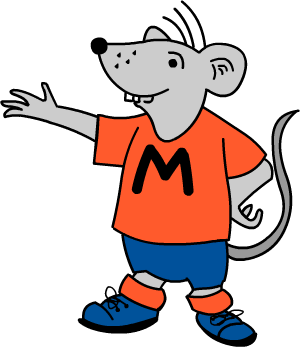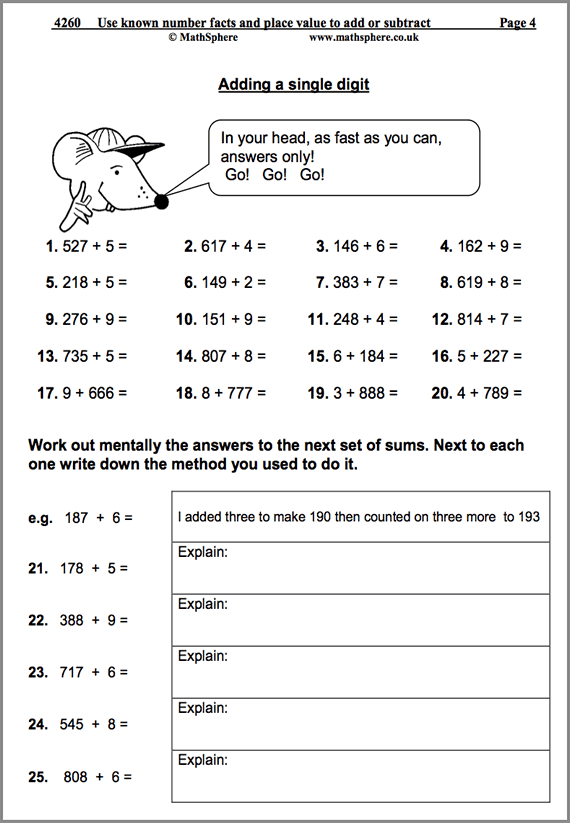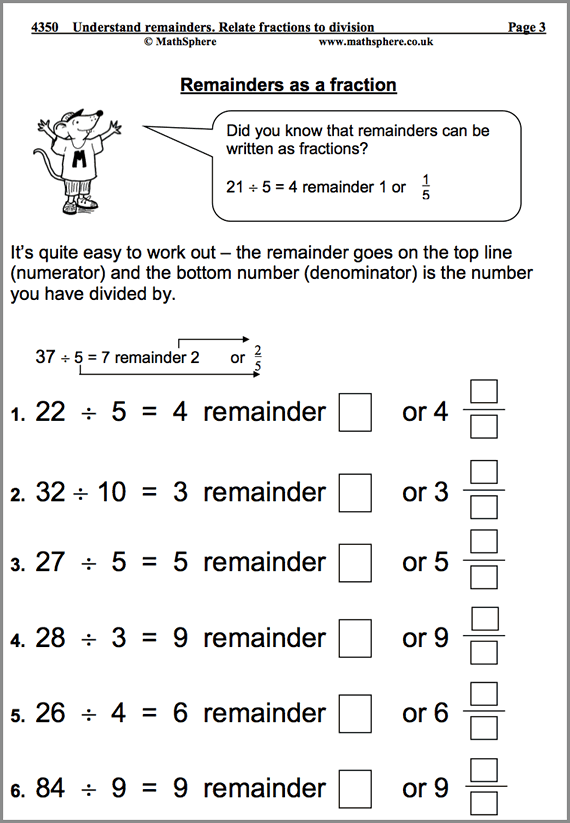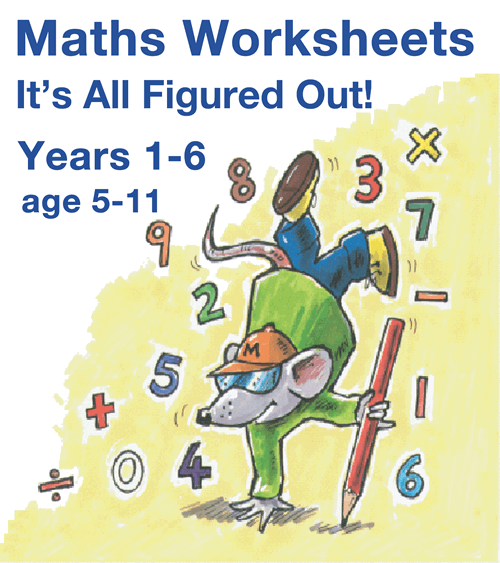# Year 4 Maths Worksheets

Price: £14.99     Digital Download

A practical and easy to use resource covering all aspects of the new 2014 Programme of Study for Year 4 (8‐9 year olds). Whether you are looking for inspiration in the classroom, homework sheets or extra support as a parent, the impressive set of MathSphere worksheets is a great solution.

## The Very Best Year 4 Maths Worksheets

A key part of Year 4 MathSphere worksheets is to help children become quick and accurate with mental arithmetic as well as improving written methods of calculating. The vast collection of resources covers all of this and much, much more.

• Updated for the very latest statutory requirements with many new pages added
• Answers included to make marking simple
• Concept pages clearly explain the ideas being taught
• Over 800 high quality pages of questions and answers
• Single user licence for teachers or parents (school site licence upgrade available)
• MathSphere has been providing superb resources on-line for more than 15 years## Try Some Free Sample Worksheets

To give you a better idea of the worksheets available we have provided a selection of free modules for Year 4.

Please download these samples, print them out and use at home, or photocopy and use in the classroom.18 Pages

#### Use Known Facts To Add & Subtract

Practice with calculating rapidly using a range of methods.19 Pages

#### Understanding Remainders

Completing division calculations by using remainders, fractions and decimal fractions.11 Pages

#### Choose Appropriate Methods of Calculating

Children make and justify decisions about how to solve number problems.

## List of modules

It is impossible to describe in a single page the massive amount of high quality maths contained in this one product.

To give you some idea, have a look at the list of all modules:

### Number and Place Value 94 pages

 Counting in multiples of 7, 8, 9 and 25. 10 Find 1, 10, 100 or 1000 more. 16 Read and write whole numbers. Partition. 14 Revise vocabulary for comparing and ordering numbers. 13 Recognise and order negative numbers. 13 Extend number sequences. 12 Revise rounding numbers. 11 Roman Numerals. 5

### Multiplication and Division 188 pages

 7x table. 12 9x table. 14 11x and 12x tables up to 12. 13 Tables: speed sheets. 21 More multiplication facts. Doubling and halving. 11 Multiply and divide mentally. 13 Multiply and divide by 10 and 100 and 1000. 16 Develop written methods of multiplication. 9 Short multiplication 2-digits by 1-digit. 8 Short multiplication 3-digits by 1-digit. 9 Revise understanding of division. 12 Understand remainders. 19 Develop written methods of division. 8 Formal method of short division with no remainders. 7 Formal method of short division with remainders. 6 Odd and even. Multiples and tests of divisibility. 10

### Measurement 87 pages

 Standard metric units of measurement. 11 Units to estimate and measure. 8 Suggest suitable measuring equipment and record results. 20 Measure and calculate the perimeter and area of simple shapes. 16 Measurement investigation. 4 Solve problems involving length, mass or capacity. 7 Using the 24-hour clock. 14 Solve problems involving time. 7

### Statistics 21 pages

 Collect, sort and organise data. Use tables, graphs and charts (1) 11 Collect, sort and organise data. Use tables, graphs and charts (2) 10

### Mental arithmetic 75 pages

 Autumn term. 25 Spring term. 25 Summer term. 25

### Addition and Subtraction 140 pages

 Revise addition and its relationship to subtraction. 15 Revise subtraction and its relationship to addition. 12 Add or subtract the nearest multiple of 10 and adjust. 13 Use the relationship between addition and subtraction. 21 Use knowledge of addition and subtraction and place value. 19 Use known number facts to add and subtract mentally. 18 Refine and use efficient written methods of addition 13 Formal written addition of 4-digit numbers. 7 Refine and use efficient written methods of subtraction. 12 Formal written subtraction of 4-digit numbers. 10

### Fractions 101 pages

 Revise equivalent fractions. 12 Count in hundredths. 4 Find fractions of numbers or quantities. 7 Identify pairs of fractions that total 1. 10 Add and subtract fractions. 12 Use decimal notation. Order decimal fractions. 12 Decimal fractions. 12 Recognise equivalence between decimals and fractions. 13 Divide by 10 and 100. 7 Solve problems involving money. 8 Round decimals. 4

### Geometry 78 pages

 Describe and classify 3D and 2D shapes. 16 Visualise 3-D objects and make nets of common solids. 10 Make turns; estimate, draw and measure angles. 18 Symmetry; reflections and translations. 16 Recognise position and direction; use co-ordinates. 13 Triangle investigation. 5

### Using and Applying Mathematics 111 pages

 Choose appropriate number operations. 11 Choose method of calculating, including using calculators. 11 Explain methods and reasoning about numbers. 8 Investigate the multiplication square. 8 Solve problems, recognise patterns, generalise and predict. 10 Solve one and two step word problems. 8 Make and investigate general statements about numbers and shapes. 10 Revise checking results of calculations. 11 Develop calculator skills 14 Maths vocabulary games. 8 Lines and rectangles investigation. 4 Palindromic numbers. 8

### Quick Details

• Over 800 worksheets
• Answers included
• Concept pages included
• For ages 8 – 9
• PDF format
• Simple download

### May We Suggest?##### It's All Figured Out!

All our worksheets together for a great saving.